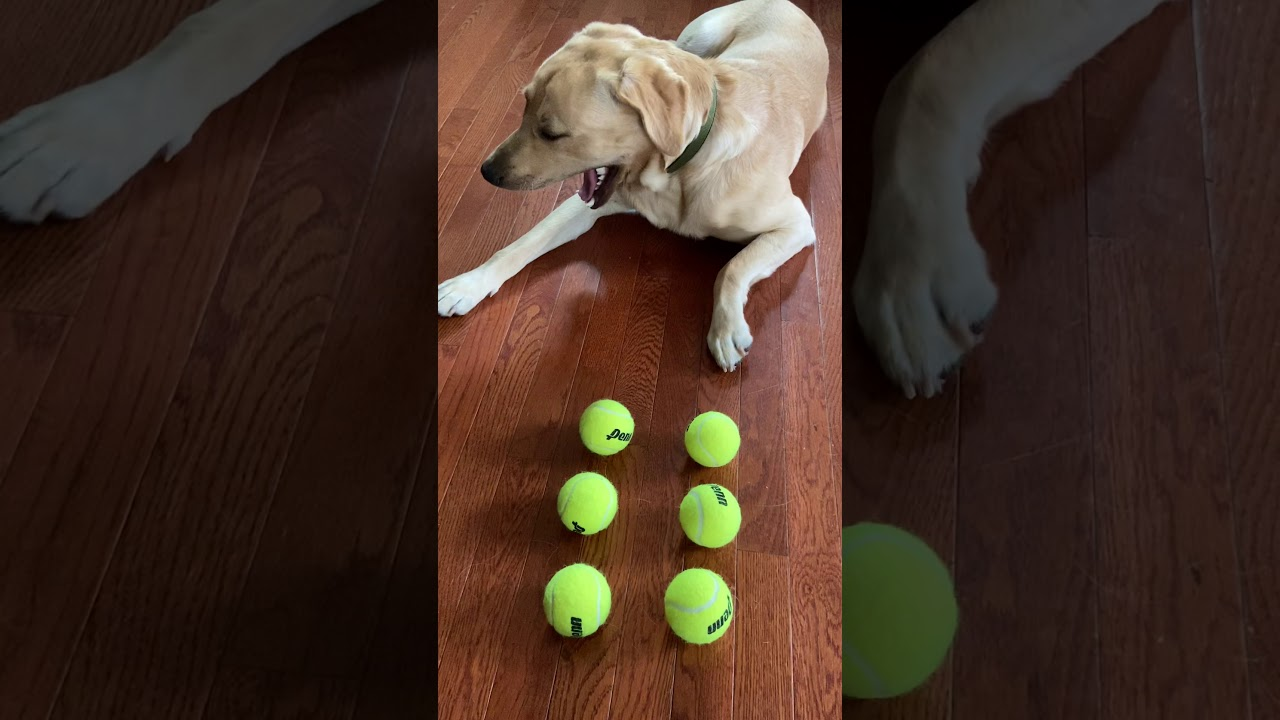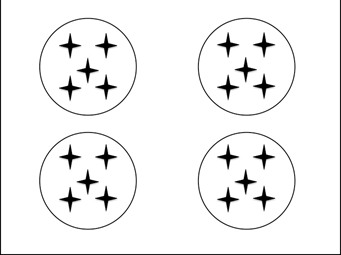Picture Model
Mystery Multiplication
Arrays
Factor or Product
100

9 x 4 = ?

36

100

Write or say the picture model in a FULL multiplication sentence.2 x 3 = 6

100

10 x 1 = ?

10

100

Write or say the array in a multiplication sentence.3 x 2

100

8 x 5 = 40

Is 5 a factor or product?

Factor

200

4 x 5 = ?

20

200

Write or say the picture model in a FULL multiplication sentence.4 x 3 = 12

200

4 x 8 = ?

32

200

Write or say the array in a multiplication sentence.3 x 3

200

6 x 2 = 12

Is 12 a factor or product?

Product

300

0 x 3 = ?

0

300

Write or say the picture model in a FULL multiplication sentence.4 x 5 = 20

300

6 x 3 = 24

True or False?

False

300

Write or say the array in a multiplication sentence.4 x 3

300

9 x 3 = 27

Is 9 a factor or product?

Factor

400

6 x 7 = ?

42

400Write or say the picture model in a FULL multiplication sentence.

3 x 5 = 15

400

9 x 2 = ?

18

400

Write or say the array in a multiplication sentence.4 x 4

400

8 x 7 = 56

What is the product in this multiplication sentence?

56

500

5 x 6 = ?

30

500

Write or say the picture model in a FULL multiplication sentence.3 x 3 = 9

500

8 x 6 = ?

48

5003 x 7

500

10 x 10 = 100

Is 100 a factor or product?

Product

Click to zoom